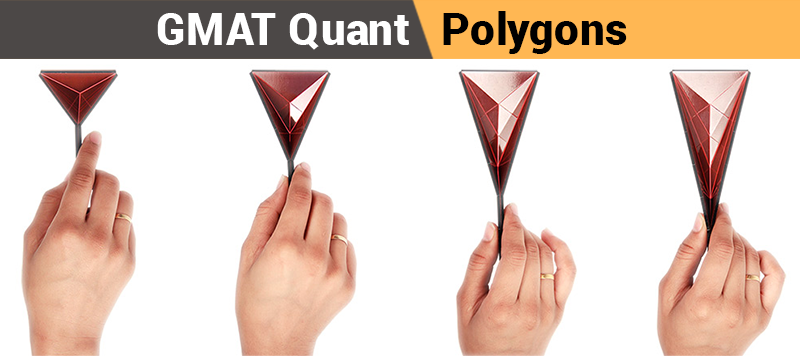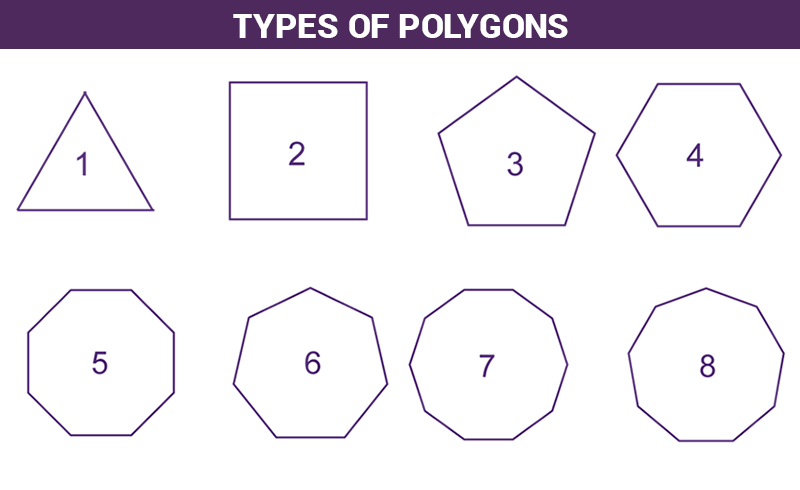# GMAT Quant: Geometry – Polygons## What is a Polygon?

Polygon is any closed plane figure that has no curvaceous sides; all its sides are straight lines. Some of the examples of a polygon are; triangles, square, rectangle, quadrilateral, pentagon, rhombus, etc.

## Properties of Polygons

• The internal angles of a polygon has a standard formula and that formula is:
The sum of all the angles in a n-sided polygon = $\small \left (n-2 \right ) \times 180^{\circ }$.
• The perimeter of a polygon = The sum of the length of all its sides

## Types of PolygonsTypes of polygons and their properties, with respect to the figure:

1. Triangle: Triangle is the most favorite figure of GMAT, The questions appearing in GMAT on the topic of triangle are not only explicitly asked about triangle but it comes with the disguise of other polygon figures as well and one can never solve it without using the concepts of triangle.
• The summation of the internal angles of a triangle is supplementary.
• The summation of any two sides will always be greater than the length of the third side, whereas, the difference of any two sides will always be less than the third side.
• The greater is the measurement of the angle of a triangle, the greater will be the length of its corresponding side.
2. Square
• All the sides of a square are equal in length as well they are spread at an angle of $\small 90^{\circ }$.
• The opposite sides in a square will always be parallel to one another.
• The diagonals of a square are equal and bisect at complementary angles.
3. Pentagon
• All sides of a pentagon are of the same length as well as all interior angles are of the same size.
• Pentagon is composed of three triangles, so the total internal angles of a pentagon = $\small 3 \times 180^{\circ} = 540^{\circ}$.
• Each angle of a pentagon is, $\small 540^{\circ} \div 5 = 180^{\circ}$.
• The central angle of a pentagon made by each side = $\small 360^{\circ} \div 5 = 72^{\circ}$.
4. Hexagon
• Hexagon is a combination of four triangles, so sum of all the internal angles = $\small 180^{\circ} \times 4 = 720^{\circ}$.
• All sides of a hexagon are of the same length as well all interior angles are of the same size.
• Each interior angle = $\small 720^{\circ} \div 6 = 120^{\circ}$.
• The central angle of a hexagon made by each side = $\small 360^{\circ} \div 6 = 60^{\circ}$.
5. Heptagon
• Heptagon is a made up of five triangles, so sum of all the internal angles = $\small 180^{\circ} \times 5 = 900^{\circ}$.
• All sides of a heptagon are of the same length as well all interior angles are of the same size.
• Each interior angle = $\small 900^{\circ} \div 7 = 128.57^{\circ}$.
• The central angle of a heptagon made by each side = $\small 360^{\circ} \div 7 = 51.43^{\circ}$.
6. Octagon
• Octagon can be divided into six triangles, so sum of all the internal angles = $\small 180^{\circ} \times 6 = 1080^{\circ}$.
• All sides of a octagon are of the same length as well all interior angles are of the same angle.
• Each interior angle = $\small 1080^{\circ} \div 8 = 135^{\circ}$.
• The central angle of a octagon made by each side = $\small 360^{\circ} \div 8 = 45^{\circ}$.

The polygons that have high degree of symmetry are known as regular polygons. For example: square, rhombus, equilateral triangle, etc.

## Solved Example

Let us solve a problem and see what standard of problem in polygon is touched in GMAT.

Question: What is the area of regular hexagon with side length of 6?Solution:

If we look at the diagram, then we can cut this regular hexagon into six equilateral triangles.

Formula for $\small Area \ of Hexagon = 6 \times Area \ of \ Triangle \times a^{2}$

Applying the formula: $\small 6 = \frac{\sqrt{3}}{4} a^{2}$ $\small = 6 \times \frac{\sqrt{3}}{4} \times 6^{2}$ $\small = 54\sqrt{3}$

Share your views by posting your comment below. You can ask for any assistance related to GMAT and MBA from us by calling us at +918884544444. You can write to us at gmat@byjus.com.